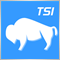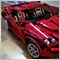# Money Flow Index: max and min values ?754

Hi all,

I'm trying to create a function that on every Bar, determines the Maximum and Minimum values of the MFI.

But it's not working. Can someone give me some hints ? Appreciated.

//---Function Max and Min values

int MFI_Period = 14;

double Max = 0;

double Min = 999999; //emulate infinite

double v_MFI;

int i = NumBars;

while(i>=0)

{

v_MFI = iMFI(NULL, 0, MFI_Period, i);

if(v_MFI > Max)

Max = v_MFI;

if(v_MFI < Min)

Min = v_MFI;

MFI_High[i] = Max;

MFI_Low[i] = Min;

i--;

}1748

JoDax:

Hi all,

I'm trying to create a function that on every Bar, determines the Maximum and Minimum values of the MFI.

But it's not working. Can someone give me some hints ? Appreciated.

You'll have to be more specific - what do you mean by "not working"?

off hand,

`int i = NumBars-1;`

I assume your NumBars is total number of bars, which you'll then have to minus 1 before you can use it to access array elements (since array idx starts from 0, not 1).

Also, your algorithm appears to be finding the min and max MFI for the ENTIRE chart, since it loops through ALL bars within the chart... is that your objective?754

Seng Joo Thio:

You'll have to be more specific - what do you mean by "not working"?

off hand,

I assume your NumBars is total number of bars, which you'll then have to minus 1 before you can use it to access array elements (since array idx starts from 0, not 1).

Also, your algorithm appears to be finding the min and max MFI for the ENTIRE chart, since it loops through ALL bars within the chart... is that your objective?

By not working I mean it's not calculating the Minimum and Maximum values as expected.

I need to calculate the Min and Max for each individual Bar.1748

JoDax:

By not working I mean it's not calculating the Minimum and Maximum values as expected.

I need to calculate the Min and Max for each individual Bar.

I did not compile your code, but

`v_MFI = iMFI(NULL, 0, MFI_Period, i);`

this line alone gets you the MFI value for bar i (which is already ONE bar) - there is no minimum or maximum for ONE bar.22610

JoDax: I need to calculate the Min and Max for each individual Bar.
1. There is no min/max for a bar. There is only the current value for a bar.
2. You could just monitor bar zero and find how it varied (min/max) but you'll find very little difference.